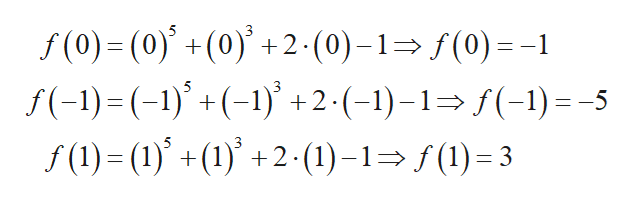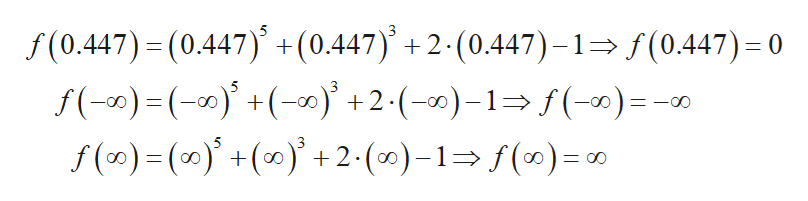Let f (x) = x5 + x3 + 2x − 1.The function f is increasing on the following interval(s): (a, b)[a, b]    (−∞, a)(−∞, a](a, ∞)[a, ∞)(−∞, a) ∪ (b, ∞)(−∞, a] ∪ [b, ∞)(−∞, ∞)Nonea =  b =   f is decreasing on the following interval(s):(c, d)[c, d]    (−∞, c)(−∞, c][c, ∞)(c, ∞)(−∞, c) ∪ (d, ∞)(−∞, c] ∪ [d, ∞)(−∞, ∞)Nonec =  d =

Question
Let f (x) = x5 + x3 + 2x − 1.

The function f is increasing on the following interval(s):

(a, b)
[a, b]

(−∞, a)
(−∞, a]
(a, ∞)
[a, ∞)
(−∞, a) ∪ (b, ∞)
(−∞, a] ∪ [b, ∞)
(−∞, ∞)
None

a =
b =

f is decreasing on the following interval(s):

(c, d)
[c, d]

(−∞, c)
(−∞, c]
[c, ∞)
(c, ∞)
(−∞, c) ∪ (d, ∞)
(−∞, c] ∪ [d, ∞)
(−∞, ∞)
None

c =
d =

Step 1

Consider the given function:

Step 2

Now in order to draw the graph, find some values for f(x).help_outlineImage Transcriptionclosef(0)-(0)+(0)+2 (0)-1(0)-1 f(-1)= (-1)() +2-(-1)-1>f(-1) = -5 f(1)=(1)+(1)+2(1)-1(3 3 fullscreen
Step 3

Further,...help_outlineImage Transcriptionclosef(0.447) (0.447) +(0.447) +2- (0.447)-1 f(0.447)= 0 f(-)( +2.()-1 (-) =- f (0)()(0+2-(0)-1 f()= 0 5 -CO 3 = OO fullscreen

Want to see the full answer?

See Solution

Want to see this answer and more?

Our solutions are written by experts, many with advanced degrees, and available 24/7

See Solution
Tagged in

Functions